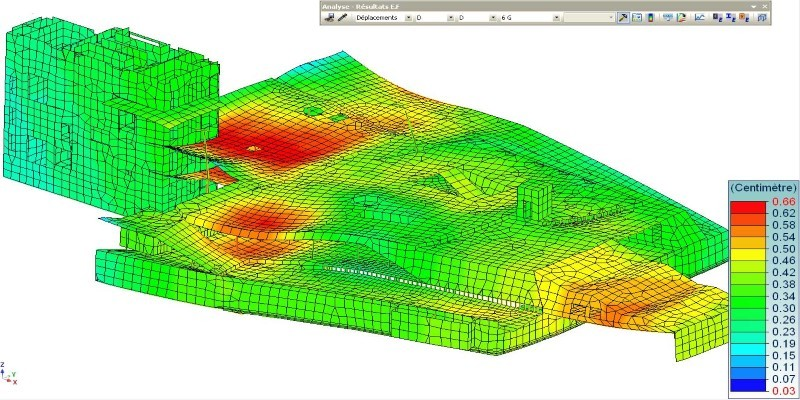# Finite Element Method: Variational Methods to Computer Programming

Wishlist Share
Share Course
Share On Social Media#### The finite Element Method (FEM) is one of the most popular numerical methods for boundary and initial value problems. One distinct feature of the Finite Element Method is that it can be generalized to the domains of any arbitrary geometry.

The theory of the Finite Element Method is developed on Variational methods. In this course, finite element formulations will be derived from the governing partial differential equation of different physical systems based on Variational methods.
It will start with one-dimensional Bar, Beam, Truss, Frame elements; and will be extended to two-dimensional structural and thermal problems. The framework of the standard master element in both 1D and 2D will be followed, so that transformation for any arbitrary geometry is well understood.
The two-dimensional formulation will be represented in the Tensorial framework, after building the necessary background in Tensor calculus. Most importantly for every element, the basic code for computer implementation will be provided and explained with step-by-step clarification. We will also elaborately present how to prepare a generalized Finite Element Method code with the first-hand implementation.

### COURSE LAYOUT

Week 1:Part1: Variational Methods:
Functional and Minimization of Functional; Derivation of Euler Lagrange equation: (a) the First variation of Functional, (b) Delta operator Functional with (a) several dependent variables, (b) higher-order derivatives; Variational statement Weak Form); Variational statement to Minimization problemRelation between Strong form, Variational statement and Minimization problem; Different approximation methods with Computer Programming: Galerkin, method, Weighted Residual method; Rayleigh-Ritz method
Week 2:Part 2. One dimensional Finite Element Analysis:
Gauss Quadrature integration rules with Computer Programming; Steps involved in Finite Element Analysis; Discrete system with linear springs; Continuous systems: Finite Element Method equation for a given differential equation Linear Element: Explaining Assembly, Solution, Post-processing with Computer Programming Quadratic element with Computer Programming: Finite element equation, Assembly, Solution, Post-processing; Comparison of Linear and Quadratic element
Week 3: Part 3. Structural Elements in One-dimensional Finite Element Method:
Bar Element with Computer Programming: Variational statement from governing differential equation; Finite element equation, Element matrices, Assembly, Solution, Post-processing; Numerical example of the conical bar under self-weight and axial point loads.Truss Element with Computer Programming: Orthogonal matrix, Element matrices, Assembly, Solution, Post-processing; Numerical example

Week 4:Beam Formulation: Variational statement from governing differential equation; Boundary terms; Hermite shape functions for beam element with Computer Programming: Finite element equation, Element matrices, Assembly, Solution, Post-processing, Implementing arbitrary distributive load; Numerical example

Week 5:Frame Element with Computer Programming: Orthogonal matrix, Finite element equation; Element matrices, Assembly, Solution, Post-processing; Numerical example
Part 4. Generalized 1D Finite Element code in Computer Programming: Step by step generalization for any no. of elements, nodes, any order Gaussian quadrature; Generalization of Assembly using connectivity data; Generalization of loading and imposition of boundary condition; Generalization of Post-processing using connectivity data;
Week 6:Part 5. Brief background of Tensor calculus: Indicial Notation: Summation convention, Kronecker delta and permutation symbol, epsilon-delta identity; Gradient, Divergence, Curl, Laplacian; Gauss-divergence theorem: different forms
Week 7 & 8: Part 6. Two-dimensional Scalar field problems:
2D Steady-State Heat Conduction Problem, obtaining weak-form, introduction to triangular and quadrilateral elements, deriving element stiffness matrix and force vector, incorporating different boundary conditions, numerical example. Computer implementation: obtaining connectivity and coordinate matrix, implementing numerical integration, obtaining global stiffness matrix and global force vector, incorporating boundary conditions, and finally post-processing.
Week 8 & 9:Part 7. Two-dimensional Vector field problems:
2D elasticity problem, obtaining the weak form, introduction to triangular and quadrilateral elements, deriving element stiffness matrix and force vector, incorporating different boundary conditions, numerical example. Iso-parametric, sub-parametric and super-parametric elements Computer implementation: a vivid layout of a generic code will be discussed Convergence, Adaptive meshing, Hanging nodes, Post-processing, Extension to three-dimensional problems Axisymmetric Problems: Formulation and numerical examples

Week 10:Part 8. Eigenvalue problems
Axial vibration of the rod (1D), formulation and implementation Transverse vibration of beams (2D), formulation and implementation
Week 11:Part 9. The transient problem in 1D & 2D Scalar Valued Problems
Transient heat transfer problems, discretization in time: method of lines and Rothe method, Formulation and Computer implementations
Week 12:Choice of solvers: Direct and iterative solvers

Finite Element Method INTENDED AUDIENCE: Final year Under Graduate Students, First-year Post Graduate Students

### What Will You Learn?

• Week 1:Part1: Variational Methods
• Week 2:Part 2. One dimensional Finite Element Analysis
• Week 3: Part 3. Structural Elements in One dimensional FEM
• Week 4:Beam Formulation
• Week 5:Frame Element with Computer Programming: Orthogonal matrix, Finite element equation; Element matrices, Assembly, Solution, Post-processing
• Week 6:Part 5. Brief background of Tensor calculus
• Week 7 & 8: Part 6. Two-dimensional Scalar field problems
• Week 8 & 9:Part 7. Two-dimensional Vector field problems:
• Week 10:Part 8. Eigenvalue problems
• Week 11:Part 9. The transient problem in 1D & 2D Scalar Valued Problems
• Week 12:Choice of solvers: Direct and iterative solvers

00:00
00:00
00:00
00:00
00:00
00:00
00:00
00:00
00:00
00:00
00:00
00:00
00:00
00:00
00:00
00:00
00:00
00:00
00:00
00:00
00:00
00:00
00:00
00:00
00:00
00:00
00:00
00:00
00:00
00:00
00:00
00:00
00:00
00:00
00:00
00:00

### Student Ratings & ReviewsNo Review Yet